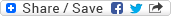## Friday, May 23, 2008

### Adobe - C code for allocating a 2D (or 3D) array

Question: Write C code to dynamically allocate and free memory for a 2D (or 3D) array.

Note: Memory-based question, suppodely a part of 'C Programming' section of the written test for a Dev position at Adobe.

Solution: Here, I'm taking the case of a 2D array only. Allocation of a 3D array will also be quite similar. Let number of rows be ROW and number of columns be COL. There are at least three ways of doing the allocation, which are:-

Method #1: Store the elements of the array as a set of ROW, each of which holds COL integers. An array of ROW integer pointers, each of which will point to the corresponding row having COL integers each. Array is accessed as arr[i][j]. The allocation may be Non-Contiguous in this case.Find above the C-code for allocating and freeing a 2D array following this method. Click the image to enlarge it.

Method #2: Store the elements of the array as a 1D array of ROW*COL integers with an array of ROW integer pointers which refer to the appropriate places in the previously allocated 1D array for the arr[i][j] type of access. In this case the allocation will always be Contiguous.Find above the C-code for allocating and freeing a 2D array following this method. Click the image to enlarge it.

Method #3: This method is just a variant of the second method. In this case we allocate memory for 1D of ROW*COL integers and access the elements as arr[COL * i + j], instead of arr[i][j]. The allocation is always Contiguous in this case as well.Find above the C-code for allocating and freeing a 2D array following this method. Click the image to enlarge it.